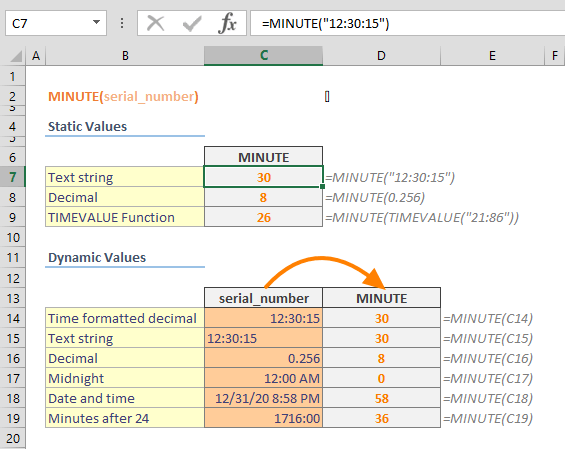The MINUTE Excel function is a Date & Time formula that returns the minute value from a specified time value. The return value is an integer, ranging from 0 to 59, and the minute values reset after 60 minutes. In this guide, we’re going to show you how to use the MINUTE Excel function and also go over some tips and error handling methods.

## Supported versions

• All Excel versions

## MINUTE Excel Function Syntax

MINUTE(serial_number)

## Arguments

 serial_number The time value that contains the minute you want to find. You can use a text string like "8:30" or a decimal number like 0.24125 which means 5:47:24 AM.

## Examples

The MINUTE Excel function only requires a single argument to execute: serial_number. Although the argument name contains "number" in its name, Excel can evaluate time values that are part of a string as well. Simply enter the serial_number as a static value, a cell reference, or a return value from another function that can generate a time value. TIME and TIMEVALUE functions can be used to provide the serial_number value.

=MINUTE("12:30:15") returns 12

=MINUTE(0.256) returns 8

=MINUTE(TIMEVALUE("28:86")) returns 26 because MINUTE resets after 60.## MINUT Excel Function Tips

• Excel stores date and time as numbers. Excel assumes that the date 1/1/1900 is “1″, and increases this number by 1 for each subsequent day. In a similar way, the time values can only be between 0 and 999999999. For example; 6:00 AM is equal to 0.25. Because of this, the TIMEVALUE function in Excel returns a number that is displayed as a time value.
• The time value is zero on 12:00 AM.
• VBA also has a function hat shares the same name. The usage is very similar to its formula counterpart.
``` Dim MyTime, MyMinute
MyTime = #4:35:17 PM#    ' Assign a time.
MyMinute = Minute(MyTime)    ' MyMinute contains 35.

You can use HOUR and SECOND functions to get hour and second values from a time value respectively.

Issues

The MINUTE Excel function returns a #NUM! error if the serial_number is negative or too big.
```